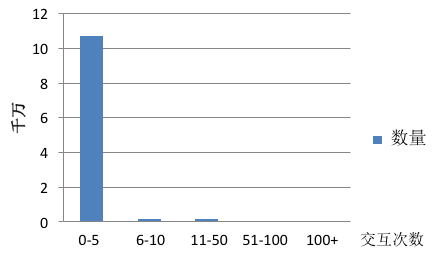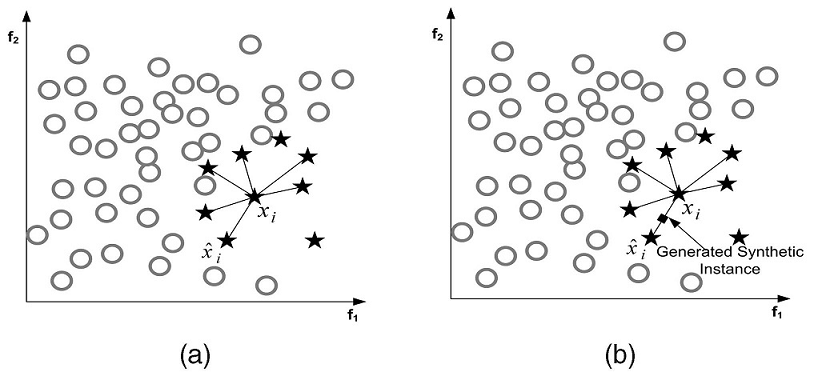## 一、数据不平衡## 二、如何解决

### 2. 数据合成Borderline-SMOTE的解决思路是寻找那些应该为之合成新样本的小众样本。即为每个小众样本计算K近邻，只为那些K近邻中有一半以上大众样本的小众样本生成新样本。直观地讲，只为那些周围大部分是大众样本的小众样本生成新样本，因为这些样本往往是边界样本。确定了为哪些小众样本生成新样本后再利用SMOTE生成新样本。

ADASYN的解决思路是根据数据分布情况为不同小众样本生成不同数量的新样本。首先根据最终的平衡程度设定总共需要生成的新小众样本数量$$G$$，然后为每个小众样本$$x_i$$计算分布比例$$\Gamma_i$$：$$\Gamma_i=\frac{\Delta_i/K}{Z}$$，其中$$\Gamma_i$$是$$x_i$$K近邻中大众样本的数量，$$Z$$用来归一化使得$$\sum\Gamma_i=1$$，最后为小众样本$$x_i$$生成新样本的个数为$$g_i=\Gamma_i\times G$$，确定个数后再利用SMOTE生成新样本。

### 3. 加权

k C(k,1) C(k,2) 0
1 2 k
1 0 C(1,2) C(1,k)
2 C(2,1) 0